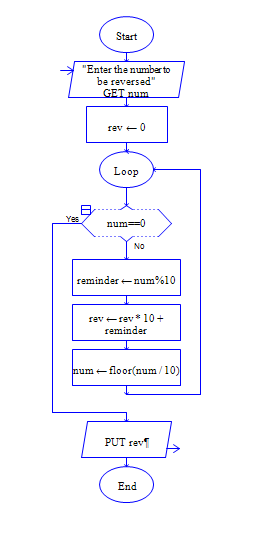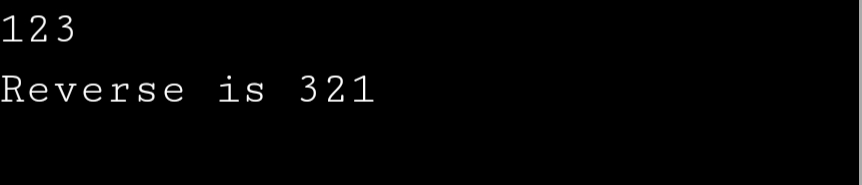# Reverse the digits of a number using Python

Get a number and reverse the digits of the number.

Sample Input 1:

Enter the number: 234

Sample Output 1:

432 is reverse of 234

#### Flow Chart Design#### Program or Solution

``` num = int(input("enter a number"))n = numreverse = 0while n != 0:    rem = n % 10    reverse = reverse * 10 + rem    n = n // 10print("{} is reverse of {}".format(reverse,num)) ```

#### Output#### Program Explanation

initialize total is equal to 0

calculate the remainder of a number by doing number % 10,  add the remainder to (total * 10) and divide the number by the 10 and repeat the above steps till number becomes zero.

Print the total, you will get the sum of digits of the number.